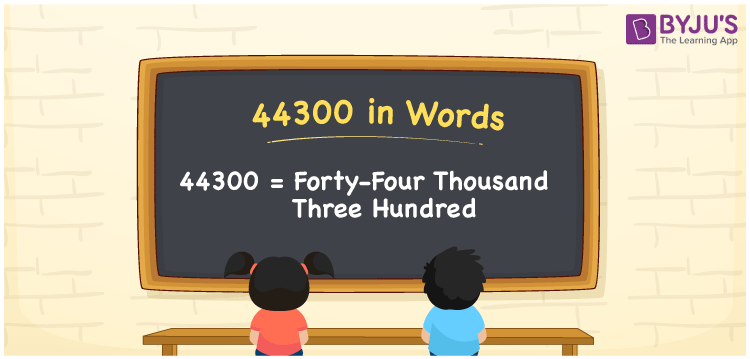# 44300 in Words

44300 in words can be written as Forty-Four Thousand Three Hundred. The article on numbers in words will provide you a clear cut knowledge of basic Maths concepts. If you deposit Rs. 44300 at the bank, then you can say that “I deposited Forty-Four Thousand Three Hundred Rupees at the bank”. Therefore, 44300 can be read as “Forty-Four Thousand Three Hundred” in words.

 44300 in words Forty-Four Thousand Three Hundred Forty-Four Thousand Three Hundred in Numbers 44300

## 44300 in English Words## How to Write 44300 in Words?

The place value chart having the details of five digits in 44300 is provided below in the form of a table for the understanding of students.

 Ten Thousands Thousands Hundreds Tens Ones 4 4 3 0 0

44300 in expanded form is:

4 x Ten Thousand + 4 x Thousand + 3 × Hundred + 0 × Ten + 0 × One

= 4 x 10000 + 4 x 1000 + 3 × 100 + 0 × 10 + 0 × 1

= 40000 + 4000 + 300

= 44300

= Forty-Four Thousand Three Hundred

Therefore, 44300 in words is written as Forty-Four Thousand Three Hundred.

44300 is a natural number that precedes 44301 and succeeds 44299.

44300 in words – Forty-Four Thousand Three Hundred

Is 44300 an odd number? – No

Is 44300 an even number? – Yes

Is 44300 a perfect square number? – No

Is 44300 a perfect cube number? – No

Is 44300 a prime number? – No

Is 44300 a composite number? – Yes

## Frequently Asked Questions on 44300 in Words

Q1

### Write 44300 in words.

44300 can be written in words as “Forty-Four Thousand Three Hundred”.
Q2

### How do you write Forty-Four Thousand Three Hundred in numbers?

Forty-Four Thousand Three Hundred can be written in numbers as 44300.
Q3

### Is 44300 an even number?

Yes, 44300 is an even number as it is completely divisible by 2.